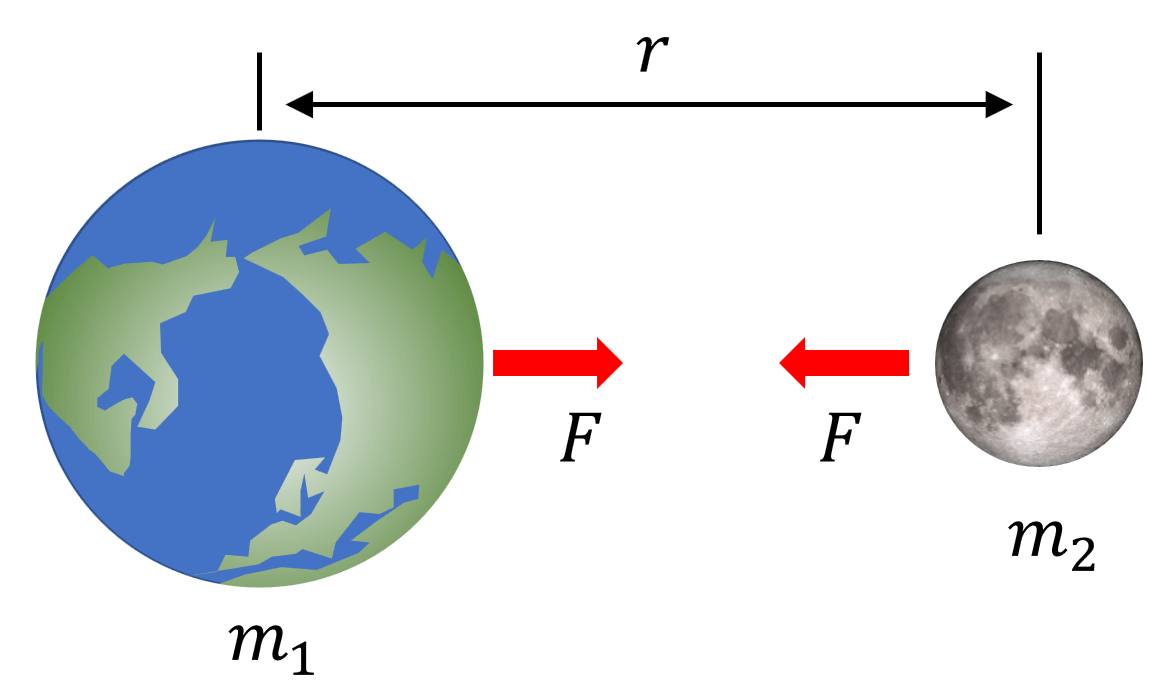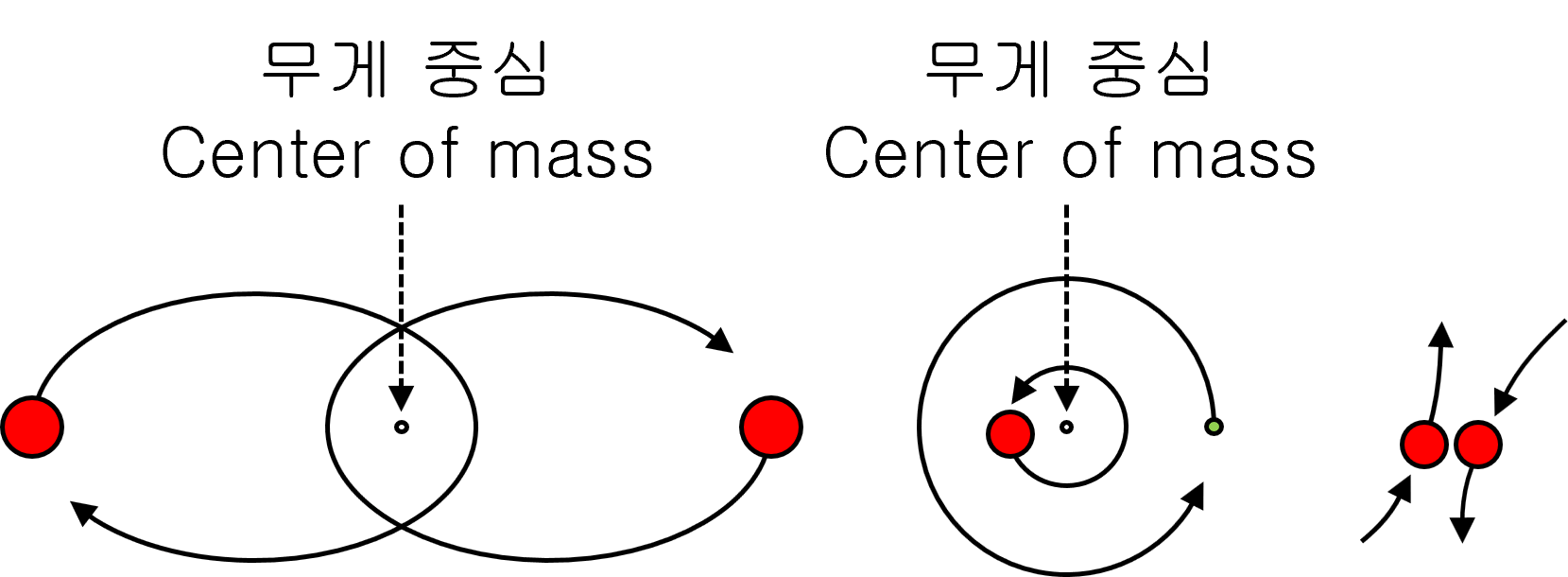# Three Body Problem

* As the operation is repeated, the above simulation may expand the error due to the decimal operation’s limitation.
* If your computer is outside of the limits that it can handle (overly large or small numbers), it may freeze.

## Two-body problem

In classical mechanics, the two-body problem is a mathematical problem of how two bodies move after a certain amount of time, given their mass, current speed, and motion direction. It is assumed that both objects move only by gravity.$F=G\frac { { m }_{ 1 }{ m }_{ 2 } }{ { r }^{ 2 } }$

Satellites that orbiting planets and binary star systems are two-body problems.## Three Body Problem

The three-body problem is a mathematical problem of how the three bodies move after knowing the mass, current speed, and movement direction.
The two-body problem is solved easily by simple equations, while the Three-Body Problem does not have a perfect mathematical solution. All the solutions presented historically are only approximate solutions.

The three-body problem began with the orbits of the three bodies of the Sun, Earth, and Moon. Isaac Newton, in his book Principia, deals with the case of three moving bodies in gravity. However, he couldn’t explain mathematically why the three heavenly bodies had a stable orbit. Since then, many have failed to solve the three-body problem. In 1890 Henri Poincaré proved it impossible to obtain a general solution to the three-body problem, which later became the basis of chaos theory.

## Many-body problem

The many-body problem is finding the motion state after giving the mass, initial position, and initial velocity of several objects.
We can only solve the Many-body problems approximately.